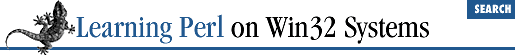home | O'Reilly's CD bookshelfs | FreeBSD | Linux | Cisco | Cisco Exam

### A.4 Chapter 5, Hashes

1. Here is one way to do it:

```%map = qw(red apple green leaves blue ocean);
print "A string please: "; chomp(\$some_string = <STDIN>);
print "The value for \$some_string is \$map{\$some_string}\n";```

The first line creates the hash, giving it the desired key-value pairs. The second line fetches a string, removing the pesky newline. The third line prints the entered value, and its mapped value.

You can also create the hash through a series of separate assignments, like so:

```\$map{'red'} = 'apple';
\$map{'green'} = 'leaves';
\$map{'blue'} = 'ocean';```
2. Here's one way to do it:

```chomp(@words = <STDIN>); # read the words, minus newlines

foreach \$word (@words) {
\$count{\$word} = \$count{\$word} + 1; # or \$count{\$word}++
}
foreach \$word (keys %count) {
print "\$word was seen \$count{\$word} times\n";
}```

The first line reads the lines into the ``` @words``` array. Recall that this method will cause each line to end up as a separate element of the array, with the newline character still intact.

The next four lines step through the array, setting ``` \$word``` equal to each line in turn. The newline is discarded with ``` chomp()``` , and then the magic comes. Each word is used as a key into a hash. The value of the element selected by the key (the word) is a count of the number of times we've seen that word so far. Initially, there are no elements in the hash, so if the word ``` wild``` is seen on the first line, we have ``` \$count{"wild"}``` , which is ``` undef``` . The ``` undef``` value plus 1 turns out to be 0 plus 1, or one. (Recall that ``` undef``` looks like a 0 if used as a number.) The next time through, we'll have 1 plus 1, or 2, and so on.

Another common way to write the increment is given in the comments. Fluent Perl programmers tend to be lazy (we call it "concise"), and would never go for writing the same hash reference on both sides of the assignment when a simple autoincrement will do.

After the words have been counted, the last few lines step through the hash by looking at each of its keys one at a time. The key and the corresponding value are printed after having been interpolated into the string.

The extra-challenge answer looks like this answer, with the ``` sort``` operator inserted just before the word ``` keys``` on the third-to-last line. Without the sorting, the resulting output is seemingly random and unpredictable. However, after being sorted, the output is predictable and consistent. (Personally, I rarely use the ``` keys``` operator without also adding a sort immediately in front of it - this method ensures that reruns over the same or similar data generate comparable results.)A.3 Chapter 4, Control StructuresA.5 Chapter 6, Basic I/O